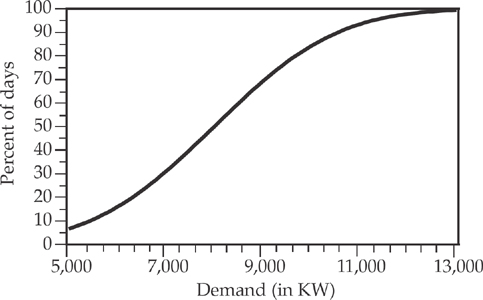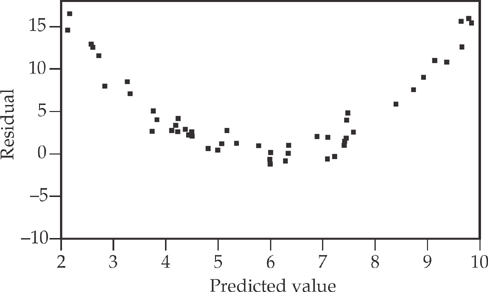# AP Statistics Practice Test 6

### Test Information10 questions23 minutes

1. Which of the following is a discrete random variable?

2. A random sample of 300 shoppers was selected to estimate the proportion of customers satisfied with the floor displays of merchandise throughout the store. What is the maximum margin of error if a 90 percent confidence interval is to be constructed?

3. Which of the following statements correctly describes the relation between a t-distribution and a standard normal distribution?

4. Researchers are concerned that the mean clutch size (number of eggs per nest) of robin’s eggs in a particular forest has decreased from the mean of 4.8 eggs measured 10 years ago. They plan to send out teams to locate and count the number of eggs in 50 different nests, and then perform a hypothesis test to determine if their concerns are well-founded. What is the most appropriate test to perform in this situation?

5. Scores on the take-home part of a test are approximately normally distributed, with a mean of 40 and a standard deviation of 3. Scores on the in-class part of the same test are also approximately normally distributed, with a mean of 34 and a standard deviation of 6. The final score on the test is the sum of the score on the take-home part and the score on the in-class part. What are the mean and standard deviation of the final test scores?

6. On a modified boxplot, an observation is classified as an outlier if

7. The following cumulative graph gives the electricity demand of a certain town.The power plant that provides electricity to this town is capable of generating 12,000 kW daily. On what percent of days will the power plant not be able to meet the demand for electricity?

8. Sixty pairs of measurements were taken at random to estimate the relation between variables X and Y. A least squares regression line was fitted to the collected data. The resulting residual plot is as follows:Which of the following conclusions is appropriate?

9. A consumer awareness group has received several complaints that the price of an asthma medicine has significantly increased in recent years. Two years ago, the mean price for this medicine was estimated to be \$78.00, and the price at different pharmacies was approximately normally distributed. The group decides to select 10 pharmacies at random and record the price of the medicine at each of the pharmacies. Assuming a 5 percent level of significance, which of the following decision rules should be used to test the hypotheses below?

H0: The mean price of medicine is \$78.00.

Ha: The mean price of medicine is higher than \$78.00.

10. A team of engineers at the research center of a car manufacturer performs crash tests to determine the proportion of times the cars’ airbags fail to operate in a crash. With the airbag system’s new modified design, the team expected to reduce the failed proportion to be below last year’s proportion of 0.08. They decided to test H0: p = 0.08 versus Ha: p < 0.08, where p = the proportion of failed airbags during crash tests. If 300 crashes performed in the lab resulted in 18 failures, which of the following is the test statistic for this test?